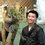# Dr. Warm's Formula for Frobenius Number

The McNugget problem originally stated that: For three different sized boxes of 6, 9, & 20 nuggets, what is the greatest number of nuggets that can’t be ordered with these combinations? For example, if you want to order 22 nuggets, the seller may put 20 nuggets in the big box with 2 remained. They may try combining the 6 and 9 combinations, but they all end up with multiples of 3, which can’t divide 22. Now it may seem that such unreachable number will be infinite, but as a matter of fact, it is not because as the number gets larger, the modular arithmetic summation between these multiples will eventually involve all factors.

For example, if you order 101 nuggets, 101 = 99 + 2. Since 99 is a multiple of 3, we have to find the number that has a remainder of 2 when divided by 3. Well, 20 is a perfect candidate!

Thus, $101 = 20 + 81 = 20 + (9 \times 9)$. That means the seller can provide you with 1 big box of 20 nuggets plus 9 boxes of 9 nuggets as your order.

This number is formally called Frobenius number written as G(a, b, c), the greatest number which can’t be written in the summation of multiples of positive integers a, b, & c: $ax_1 + bx_2 + cx_3 = G$ G will have no solutions for non-negative integers xi.

The modified Frobenius number $F(a, b, c) = G(a, b, c) + a + b + c$

By using Johnson’s formula , $F(da, db, c) = d\cdot F(a, b, c)$

Then if $c|lcm(a,b)$ and $gcd(a, b, c) = 1$, then $a = pr; b = qs; c = rs$, for some integers p, q, r, s.

Thus, $F(a, b, c) = r[F(p, qs, s)] = rs[F(p, q, 1)]$

$F(p, q, 1) = G(p, q, 1) + p + q + 1$

By definition, $G(p, q, 1) = px_1 + qx_2 + x_3$, but since x3 can be any non-negative integer, G will always in the range from 0 to infinity. Therefore, the greatest integer, which can’t be written in non-negative summation is -1, the greatest negative integer. Hence, $F(p, q, 1) = G(p, q, 1) + p + q + 1 = -1 + p + q + 1 = p + q$

Then $F(a, b, c) = rs[F(p, q, 1)] = rs(p + q) = prs + qrs$

Note that lcm is the largest common multiple: $lcm(a, c) = prs$ and $lcm(b, c) = qrs$. Thus, $F(a, b, c) = lcm(a, c) + lcm(b, c)$.

Finally, $G(a, b, c) = F(a, b, c) -a -b -c = lcm(a, c) + lcm(b, c) -a -b -c$

I called it: Dr. Warm’s Formula.

Now let’s back to our Mcnugget question: What is the value of $G(6, 9, 20)$?

Then $lcm(9, 20) = 180$, and clearly 6 can divide 180. So let’s calculate with Dr. Warm’s formula:

$G(6, 9, 20) = lcm(6, 20) + lcm(6, 9) -6 -9 -20 = 60 + 18 -35 = 43$.

As a result, the 43 nuggets are the largest amount that can’t be ordered as the combinations of boxes as mentioned above.

Mystery solved!

Dr. Warm's Formula

If $\text{gcd}(a, b, c)= 1$ and $c|\text{lcm}(a,b)$, then $G(a, b, c) = \text{lcm}(a,c) + \text{lcm}(b,c) -a -b -c$.Note by Worranat Pakornrat
4 years, 1 month ago

This discussion board is a place to discuss our Daily Challenges and the math and science related to those challenges. Explanations are more than just a solution — they should explain the steps and thinking strategies that you used to obtain the solution. Comments should further the discussion of math and science.

When posting on Brilliant:

• Use the emojis to react to an explanation, whether you're congratulating a job well done , or just really confused .
• Ask specific questions about the challenge or the steps in somebody's explanation. Well-posed questions can add a lot to the discussion, but posting "I don't understand!" doesn't help anyone.
• Try to contribute something new to the discussion, whether it is an extension, generalization or other idea related to the challenge.
• Stay on topic — we're all here to learn more about math and science, not to hear about your favorite get-rich-quick scheme or current world events.

MarkdownAppears as
*italics* or _italics_ italics
**bold** or __bold__ bold
- bulleted- list
• bulleted
• list
1. numbered2. list
1. numbered
2. list
Note: you must add a full line of space before and after lists for them to show up correctly
paragraph 1paragraph 2

paragraph 1

paragraph 2

[example link](https://brilliant.org)example link
> This is a quote
This is a quote
    # I indented these lines
# 4 spaces, and now they show
# up as a code block.

print "hello world"
# I indented these lines
# 4 spaces, and now they show
# up as a code block.

print "hello world"
MathAppears as
Remember to wrap math in $$ ... $$ or $ ... $ to ensure proper formatting.
2 \times 3 $2 \times 3$
2^{34} $2^{34}$
a_{i-1} $a_{i-1}$
\frac{2}{3} $\frac{2}{3}$
\sqrt{2} $\sqrt{2}$
\sum_{i=1}^3 $\sum_{i=1}^3$
\sin \theta $\sin \theta$
\boxed{123} $\boxed{123}$

## Comments

Sort by:

Top Newest

brillant!!!!!!!

- 2 years, 11 months ago

Log in to reply

Thanks. :)

- 2 years, 11 months ago

Log in to reply

×

Problem Loading...

Note Loading...

Set Loading...